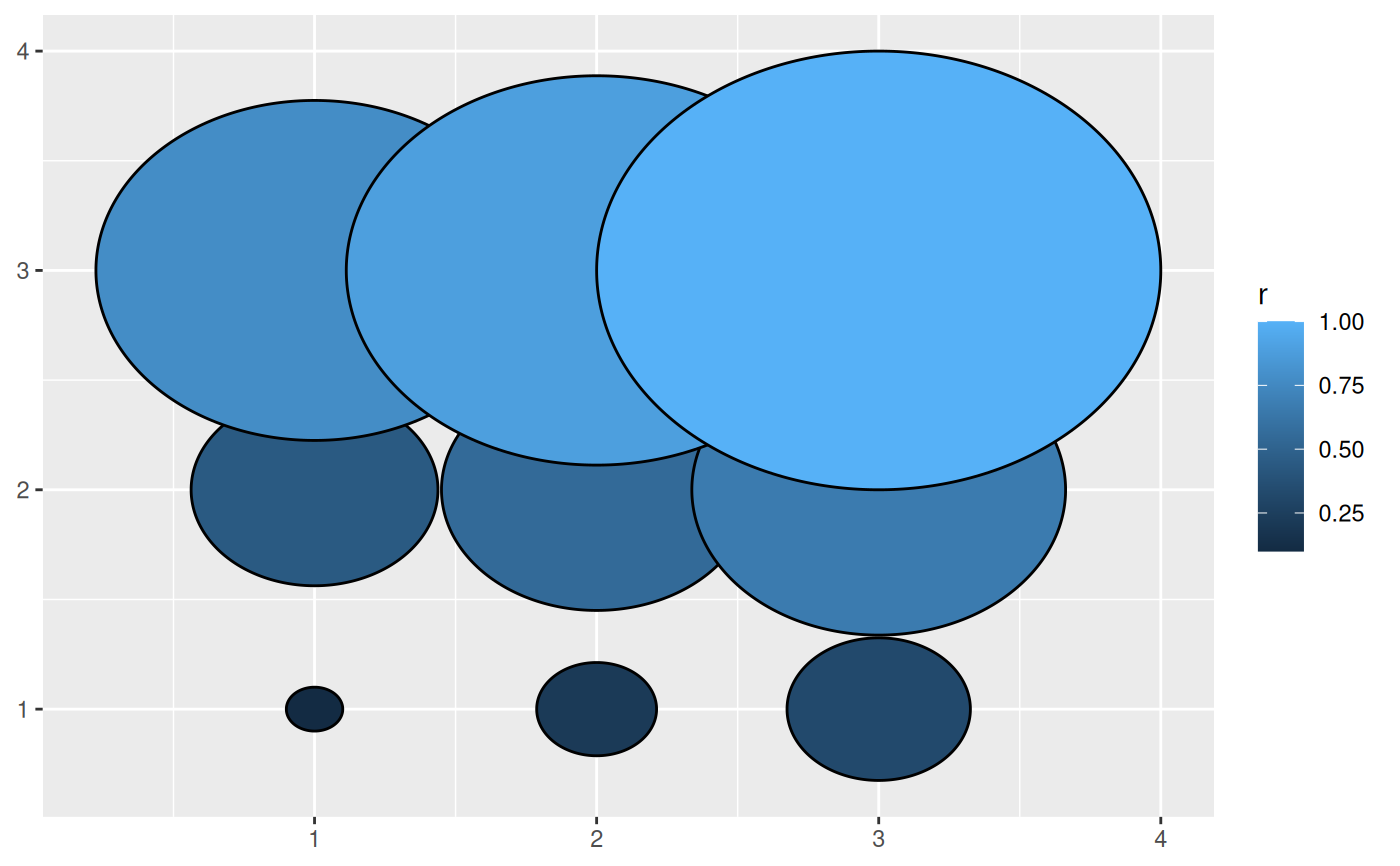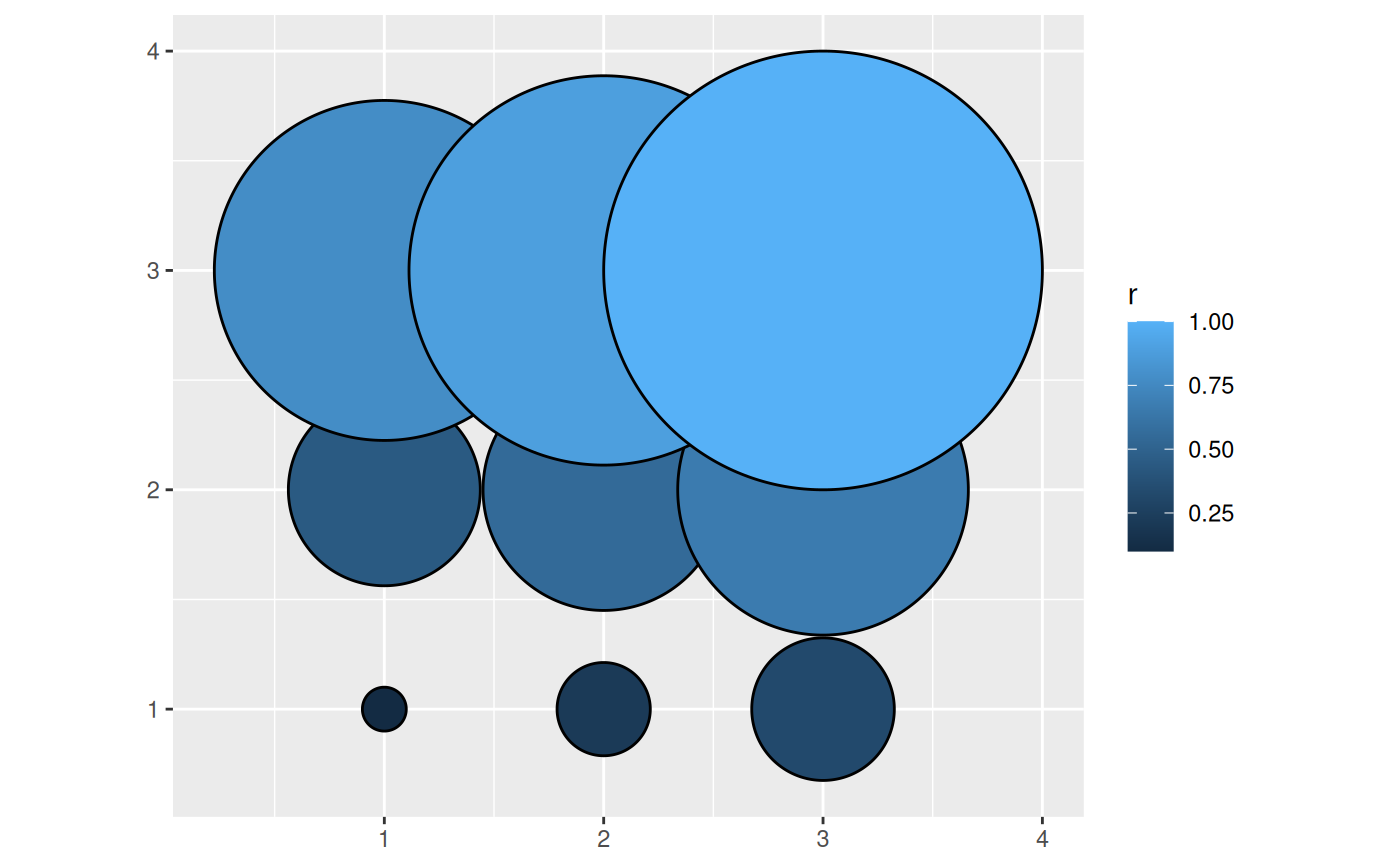This set of stats and geoms makes it possible to draw circles based on a center point and a radius. In contrast to using ggplot2::geom_point(), the size of the circles are related to the coordinate system and not to a separate scale. These functions are intended for cartesian coordinate systems and will only produce a true circle if ggplot2::coord_fixed() is used.

stat_circle(
mapping = NULL,
data = NULL,
geom = "circle",
position = "identity",
n = 360,
na.rm = FALSE,
show.legend = NA,
inherit.aes = TRUE,
...
)

geom_circle(
mapping = NULL,
data = NULL,
stat = "circle",
position = "identity",
n = 360,
expand = 0,
na.rm = FALSE,
show.legend = NA,
inherit.aes = TRUE,
...
)

## Arguments

mapping

Set of aesthetic mappings created by aes(). If specified and inherit.aes = TRUE (the default), it is combined with the default mapping at the top level of the plot. You must supply mapping if there is no plot mapping.

data

The data to be displayed in this layer. There are three options:

If NULL, the default, the data is inherited from the plot data as specified in the call to ggplot().

A data.frame, or other object, will override the plot data. All objects will be fortified to produce a data frame. See fortify() for which variables will be created.

A function will be called with a single argument, the plot data. The return value must be a data.frame, and will be used as the layer data. A function can be created from a formula (e.g. ~ head(.x, 10)).

geom

The geometric object to use to display the data, either as a ggproto Geom subclass or as a string naming the geom stripped of the geom_ prefix (e.g. "point" rather than "geom_point")

position

Position adjustment, either as a string naming the adjustment (e.g. "jitter" to use position_jitter), or the result of a call to a position adjustment function. Use the latter if you need to change the settings of the adjustment.

n

The number of points on the generated path per full circle.

na.rm

If FALSE, the default, missing values are removed with a warning. If TRUE, missing values are silently removed.

show.legend

logical. Should this layer be included in the legends? NA, the default, includes if any aesthetics are mapped. FALSE never includes, and TRUE always includes. It can also be a named logical vector to finely select the aesthetics to display.

inherit.aes

If FALSE, overrides the default aesthetics, rather than combining with them. This is most useful for helper functions that define both data and aesthetics and shouldn't inherit behaviour from the default plot specification, e.g. borders().

...

Other arguments passed on to layer(). These are often aesthetics, used to set an aesthetic to a fixed value, like colour = "red" or size = 3. They may also be parameters to the paired geom/stat.

stat

The statistical transformation to use on the data for this layer, either as a ggproto Geom subclass or as a string naming the stat stripped of the stat_ prefix (e.g. "count" rather than "stat_count")

expand

A numeric or unit vector of length one, specifying the expansion amount. Negative values will result in contraction instead. If the value is given as a numeric it will be understood as a proportion of the plot area width.

As expand but specifying the corner radius.

## Note

If the intend is to draw a bubble chart then use ggplot2::geom_point() and map a variable to the size scale

## Aesthetics

geom_circle understand the following aesthetics (required aesthetics are in bold):

• x0

• y0

• r

• color

• fill

• linewidth

• linetype

• alpha

• lineend

## Computed variables

x, y

The start coordinates for the segment

geom_arc_bar() for drawing arcs with fill

## Examples

# Lets make some data
circles <- data.frame(
x0 = rep(1:3, 3),
y0 = rep(1:3, each = 3),
r = seq(0.1, 1, length.out = 9)
)

# Behold some circles
ggplot() +
geom_circle(aes(x0 = x0, y0 = y0, r = r, fill = r), data = circles)# Use coord_fixed to ensure true circularity
ggplot() +
geom_circle(aes(x0 = x0, y0 = y0, r = r, fill = r), data = circles) +
coord_fixed()